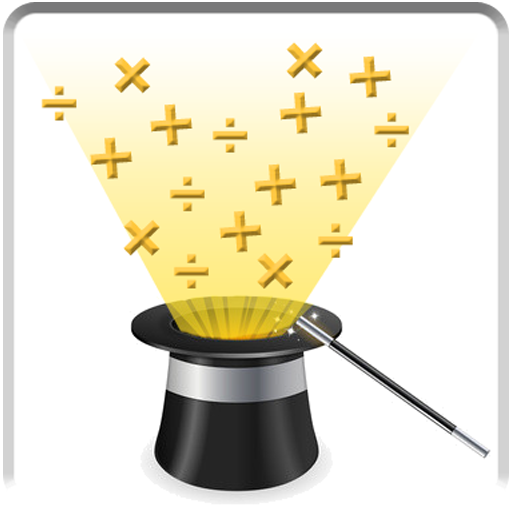# 31 Aug 2018 Game Of The Day

## Tricky Maths

### by Rohit Porwal

Free
1504
Categories Tags
###### Description

Tricky Maths program is dedicated to improve your mental calculation skills.

This program has lots of games, which will improve your calculation speed, while giving a nice brain training.

And it has many lessons on math tricks and shortcuts, which will improve enhance your mathematical skills. Overall, this program will increase your aptitude which can increase your performance in school, in college and even in your day to day life. Why should it be necessary to use your calculator for simple calculations of daily life??

It has following mathematical brain Games:
Brain Twister: Keep track of calculation and maintain your focus.

True or False: In this game, identify whether an equation is true or false.

Make the order!!: Arrange numbers in increasing or decreasing order.

Odd one out: Identify the number which is distinct from other numbers in the given set of numbers.

Match Maker: Match the numbers in pairs.

Memory Game: Remember the numbers in ascending order and then identify them in order.

Percentages: Game dedicated to questions relating to percentages.

More or Less!!: Identify whether current number is more than or less than previously shown number.

Survival: Solve the question before time ends!!

Find a question?: Find the equation which has the shown result.

Speed-o-racy: Its all about speed and accuracy.

Series: Identify the missing number in given series. Identify the pattern!!

Easy Mode: Easy questions related to addition, subtraction, multiplication and division.

Normal Mode: relatively difficult questions relating to numerous operations like squares, square roots, cubes etc.

Expert Mode: starts from level 4 of normal mode and include percentages

Addition Master:Select the numbers from the grid which will sum up to displayed number.

Spot the number: Identify the numbers as per the hint given.

Missing Signs: Identify the missing operator in the given equation.

Equal or not: Identify whether two equations will have same result or not.

Fill in the Blanks: Fill in the missing number in the given equation.

And It has following mathematical tricks lessons:
1. Multiply by 5, 10, 25 etc.
2. Vedic Multiplication
3. Multiply by 11, xx, and 111..1
4. Multiply by 12 to 19 and 112 to 119
5. Multiply by 999...9
6. Multiplication of numbers near 100
7. Multiplication of numbers near 100..0
8. Multiply 2 numbers near 50
9. Multiplication of 3 numbers near 100.
10. Multiplication of 2 no. where last 2 digits sum to 10
11. Square of no. ending with 5 or 0
12. Square of no near 50, 100, and 200
13. Square of any 2-digit no.
14. Squares of no. ending with 1, 2, 3, 4
15. Squares of no. ending with 9, 8, 7, 6
16. Square of 3-digit number
17. square root tricks
18. multiplication trick where difference is even
19. cube of numbers near 100
20. cube and cube roots
21. Divisibility rules: Test of divisibility by 2, 3, 4, 5, 6 ,7, 8, 9, 10, 11, 13, 15, 17, 19, 25

These kind of mathematical tricks will increase your calculation speed in aptitude exams which generally contains simple math questions, like as in staff selection commission(ssc) exam, IAS, PCS exam or GMAT or CAT exams. It is very crucial to solve these kind of arithmetic questions and that too with speed and accuracy.

Chart library used:
MPAndroidChart (by Philipp Jahoda)

904

1756
• GOTD

562